# Oryza Sativa Whole Network

Whole cellular network data

Whole cellular network data for Oryza Sativa

## Examples

### Basic Examples

Retrieve the graph:

 In:=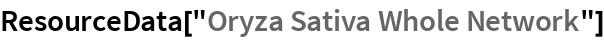Out=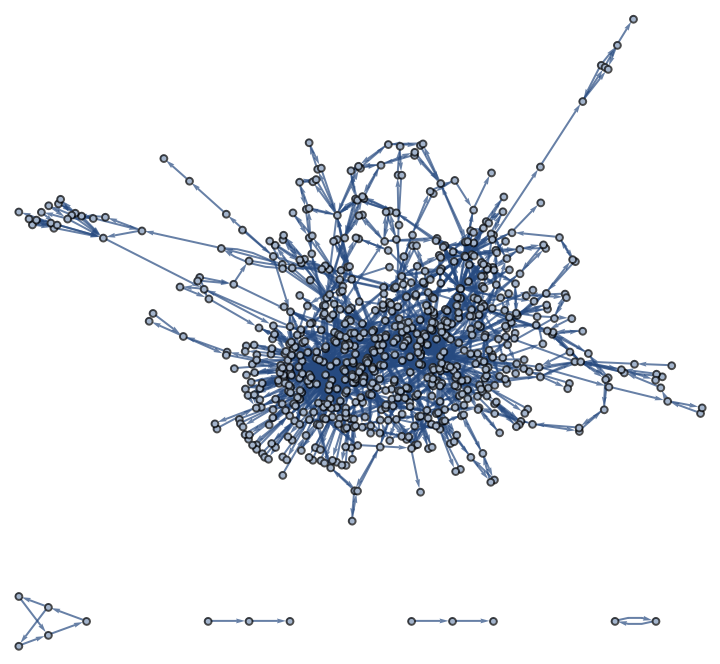Summary properties:

 In:=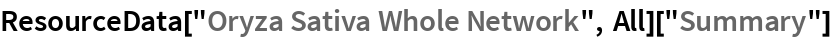Out=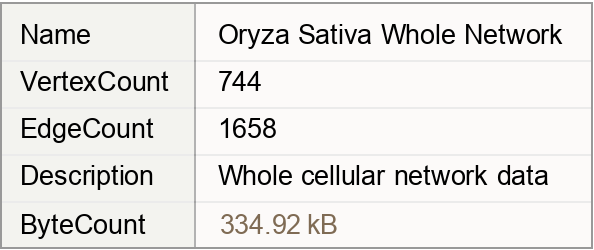### Basic Applications

Create a histogram of the centrality:

 In:=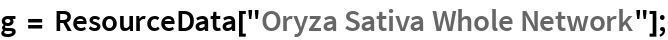In:=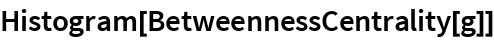Out=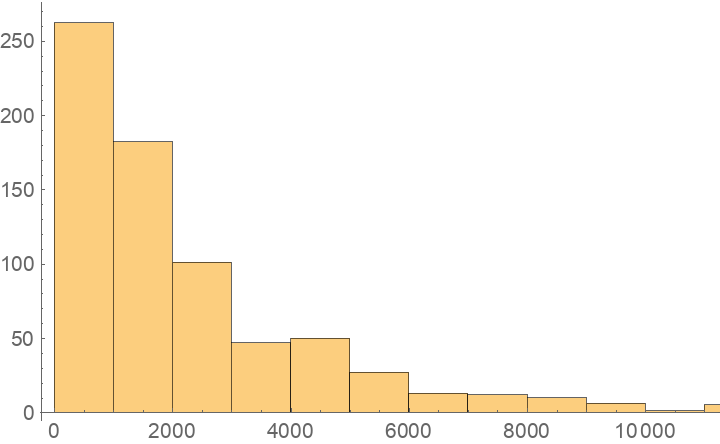Show a table of properties:

 In:=Out=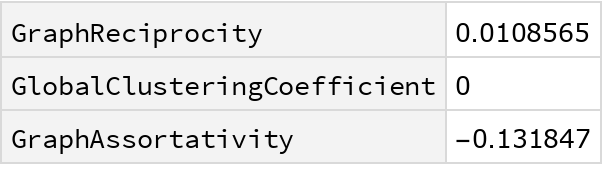Wolfram Research, "Oryza Sativa Whole Network" from the Wolfram Data Repository (2019)